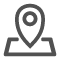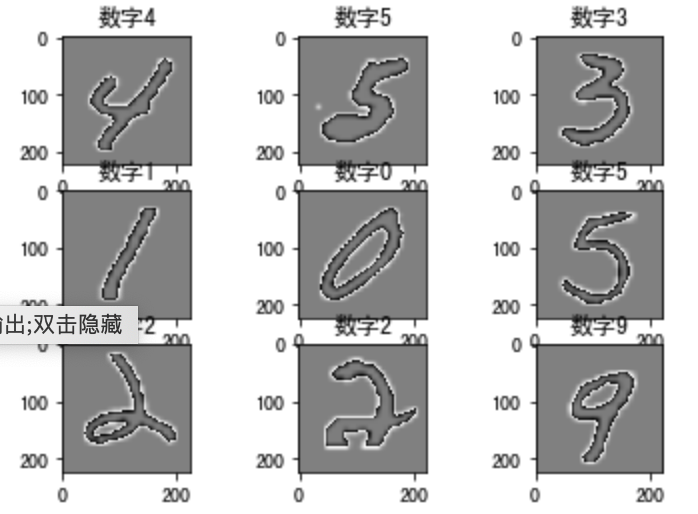# AI人工智能培训：AlexNet手写数字图像识别

## 图像分类AlexNet完手写数字势识别

2012年，AlexNet横空出世，该模型的名字源于论文第一作者的姓名Alex Krizhevsky 。AlexNet使用了8层卷积神经网络，以很大的优势赢得了ImageNet 2012图像识别挑战赛。它首次证明了学习到的特征可以超越手工设计的特征，从而一举打破计算机视觉研究的方向。

```import numpy as np
# 获取手写数字数据集
(train_images, train_labels), (test_images, test_labels) = mnist.load_data()
# 训练集数据维度的调整：N H W C
train_images = np.reshape(train_images,(train_images.shape,train_images.shape,train_images.shape,1))
# 测试集数据维度的调整：N H W C
test_images = np.reshape(test_images,(test_images.shape,test_images.shape,test_images.shape,1))```

```# 定义两个方法随机抽取部分样本演示# 获取训练集数据def get_train(size):
# 随机生成要抽样的样本的索引
index = np.random.randint(0, np.shape(train_images), size)
# 将这些数据resize成227*227大小
# 返回抽取的
return resized_images.numpy(), train_labels[index]# 获取测试集数据 def get_test(size):
# 随机生成要抽样的样本的索引
index = np.random.randint(0, np.shape(test_images), size)
# 将这些数据resize成227*227大小
# 返回抽样的测试样本
return resized_images.numpy(), test_labels[index]```

```# 获取训练样本和测试样本
train_images,train_labels = get_train(256)
test_images,test_labels = get_test(128)```

```# 数据展示：将数据集的前九个数据集进行展示for i in range(9):
plt.subplot(3,3,i+1)
# 以灰度图显示，不进行插值
plt.imshow(train_images[i].astype(np.int8).squeeze(), cmap='gray', interpolation='none')
# 设置图片的标题：对应的类别
plt.title("数字{}".format(train_labels[i]))```